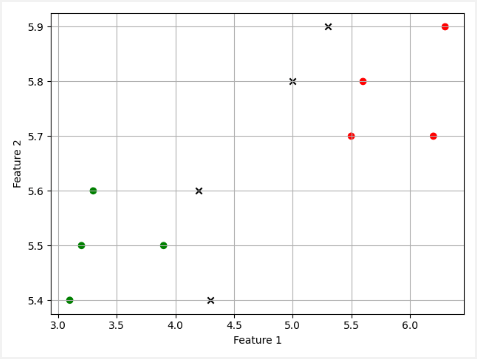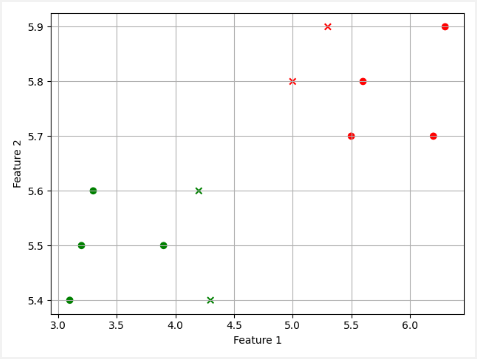# Binary Classification using TensorFlow 2

Binary classification is the process that is used to classify data points into one of two classes. For example, whether a customer will buy a product or not, emails are spam or not, whether a patient has certain disease or not.

Let’s say we have a set of labeled points:

We have two features for each point, represented by the `f1` and `f2`. In addition, we have one of two class labels for each point. Label 0 represents the first class, and label 1 represents the second class.

And we have some unlabeled points:

A trained model should predict whether a new point should be labeled 0 or 1.

This tutorial shows an example how to create and train a model which classifies data points into one of two classes. We will use TensorFlow 2.

Using `pip` package manager, install `tensorflow` from the command line.

``pip install tensorflow``

In order to better understand the data, we will display a scatter plot where each value in the data is represented by a dot. We declare arrays of features (`trainF1`, `trainF2`) and labels (`trainLabels`) for training a model. Unlabeled points for testing are declared as `testF1` and `testF2`.

plot.py

``````import numpy as np
import matplotlib.pyplot as plt

trainF1 = np.array([3.2, 6.3, 6.2, 3.3, 3.1, 5.6, 3.9, 5.5], dtype=float)
trainF2 = np.array([5.5, 5.9, 5.7, 5.6, 5.4, 5.8, 5.5, 5.7], dtype=float)
trainLabels = np.array([1, 0, 0, 1, 1, 0, 1, 0], dtype=float)

testF1 = np.array([4.3, 5.3, 5.0, 4.2], dtype=float)
testF2 = np.array([5.4, 5.9, 5.8, 5.6], dtype=float)

colors = ['red', 'green']
colorsList = [colors[int(label)] for label in trainLabels]

plt.scatter(trainF1, trainF2, c=colorsList)
plt.scatter(testF1, testF2, c='black', marker='x')
plt.xlabel('Feature 1')
plt.ylabel('Feature 2')
plt.grid()
plt.show()``````

Label 0 is represented by red dots and label 1 is represented by green dots. Unlabeled points are represented by black crosses.We build a model with three `Dense` layers. We have two features, so model has two inputs. Model has one output.

Inputs for the model should be presented in the single array. So `trainF1` and `trainF2` arrays are joined along a new axis by using the `stack` method.

The model is compiled using binary cross-entropy loss function and Adam optimizer. We use 300 epochs to train the model. After training, the model is saved in `HDF5` format.

train.py

``````from tensorflow import keras
import numpy as np

trainF1 = np.array([3.2, 6.3, 6.2, 3.3, 3.1, 5.6, 3.9, 5.5], dtype=float)
trainF2 = np.array([5.5, 5.9, 5.7, 5.6, 5.4, 5.8, 5.5, 5.7], dtype=float)
trainFeatures = np.stack((trainF1, trainF2), 1)
trainLabels = np.array([1, 0, 0, 1, 1, 0, 1, 0], dtype=float)

model = keras.Sequential([
keras.layers.Dense(64, activation='relu', input_shape=(2,)),
keras.layers.Dense(32, activation='relu'),
keras.layers.Dense(1, activation='sigmoid')
])

model.fit(trainFeatures, trainLabels, epochs=300)

model.save('model.h5')``````

A model was trained, and now we can predict labels for the given data points.

The model has one output, which value is in the range from 0 to 1. If the value of the output is less than 0.5 are assigned to label 0 and value greater than or equal to 0.5 are assigned to label 1.

• If prediction < 0.5 then label = 0
• If prediction >= 0.5 then label = 1

test.py

``````from tensorflow import keras
import numpy as np
import matplotlib.pyplot as plt

trainF1 = np.array([3.2, 6.3, 6.2, 3.3, 3.1, 5.6, 3.9, 5.5], dtype=float)
trainF2 = np.array([5.5, 5.9, 5.7, 5.6, 5.4, 5.8, 5.5, 5.7], dtype=float)
trainLabels = np.array([1, 0, 0, 1, 1, 0, 1, 0], dtype=float)

testF1 = np.array([4.3, 5.3, 5.0, 4.2], dtype=float)
testF2 = np.array([5.4, 5.9, 5.8, 5.6], dtype=float)
testFeatures = np.stack((testF1, testF2), 1)

predictedLabels = model.predict(testFeatures)
predictedLabels = [int(label >= 0.5) for label in predictedLabels]

colors = ['red', 'green']
colorsList = [colors[int(label)] for label in trainLabels]
colorsListPredicted = [colors[label] for label in predictedLabels]

plt.scatter(trainF1, trainF2, c=colorsList)
plt.scatter(testF1, testF2, c=colorsListPredicted, marker='x')
plt.xlabel('Feature 1')
plt.ylabel('Feature 2')
plt.grid()
plt.show()``````

A scatter plot shows that labels for data points were predicted correctly.